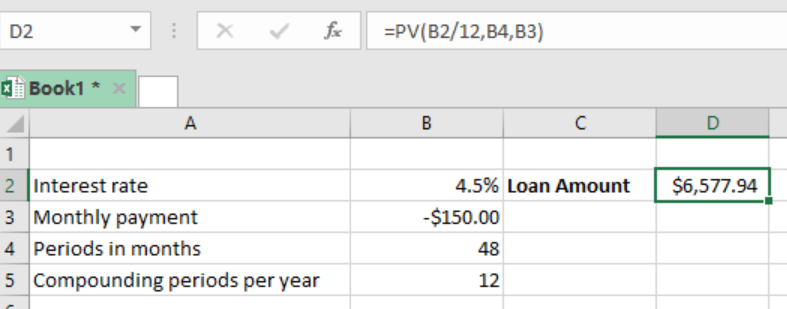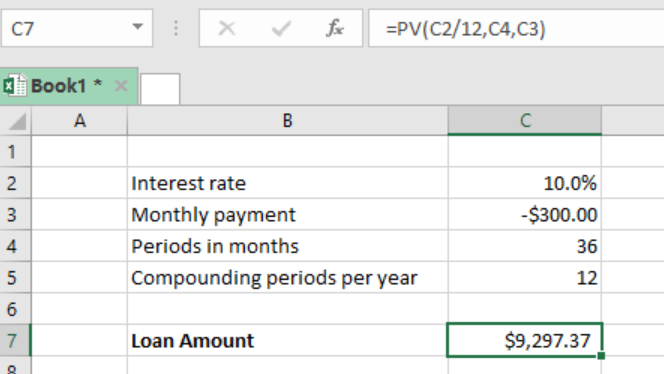Get instant live expert help with Excel or Google Sheets“My Excelchat expert helped me in less than 20 minutes, saving me what would have been 5 hours of work!”

#### Post your problem and you’ll get Expert help in seconds.

Your message must be at least 40 characters
Our professional Expert are available now. Your privacy is guaranteed.

# Excel PV Function

You can use the Excel PV function to get the present value of an investment. The function is most fundamental when it comes to calculating the value in today’s dollars of a series of future payments. While working with this function, we assume that future payments are periodic, constant with a constant rate of interest. This article will walk through the PV function.Figure 1: Getting present value using PV function

## General syntax of the formula

`=PV (rate, nper, pmt, [fv], [type])`

Where;

•         Rate– is the rate pee period, which is annual
•         Nper- total number of payment periods
•         Pmt– payment per period
•         Fv– cash balance to attain after last payment. It is optional and if omitted, it is assumed to be zero.
•         Type– when payments are due. At the end, =0 and 1 at the beginning. Default is 0.

## Understanding the Excel PV function

We use the Excel PV function to get the present value of an investment. When the above formula is applied to a set of data, it will return the present value.

The present value is the value in today’s dollars of a series of payments to be made in future. When calculating the present value, three assumptions are made:

•         Payments are periodic
•         The payments are constant i.e. they do not vary
•         The interest rate is also constant

## ExampleFigure 2: Finding the present value of future payments using PV function

In this example, our interest rate is 10%, monthly payment is \$300, and period is 36 months. Note that the interest rate is in terms of years, so we need to divide it by 12 to get it in months.

When we enter the formula `=PV (C2/12, C4, C3) `and press enter, we get the present value.

## Instant Connection to an Expert through our Excelchat Service

Most of the time, the problem you will need to solve will be more complex than a simple application of a formula or function. If you want to save hours of research and frustration, try our live Excelchat service! Our Excel Experts are available 24/7 to answer any Excel question you may have. We guarantee a connection within 30 seconds and a customized solution within 20 minutes.

### Did this post not answer your question? Get a solution from connecting with the expert.Another blog reader asked this question today on Excelchat:
Solution exampleshow would i do this question on excel? An investment will generate Â£15,000 a year for 20 years. If you require a rate of compensation of 10% and the investment costs Â£100,000, show whether it is worth buying? If expected inflation is instead estimated to be 2% higher, show how this impacts on your recommendation.
Solved by V. W. in 60 mins## Subscribe to Excelchat.coAnother blog reader asked this question today on Excelchat: